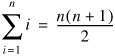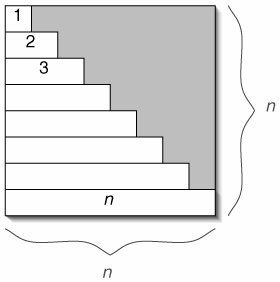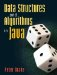# C.3. Sum of First n Integers

Here is the formula for the sum of the first n integers.This is slightly more than n2/2, as illustrated in Figure C-2.

Figure C-2. The sum of the first n integers occupies just over half of an n x n square.### C 4 Sums of Halves and DoublesData Structures and Algorithms in Java
ISBN: 0131469142
EAN: 2147483647
Year: 2004
Pages: 216
Authors: Peter Drake